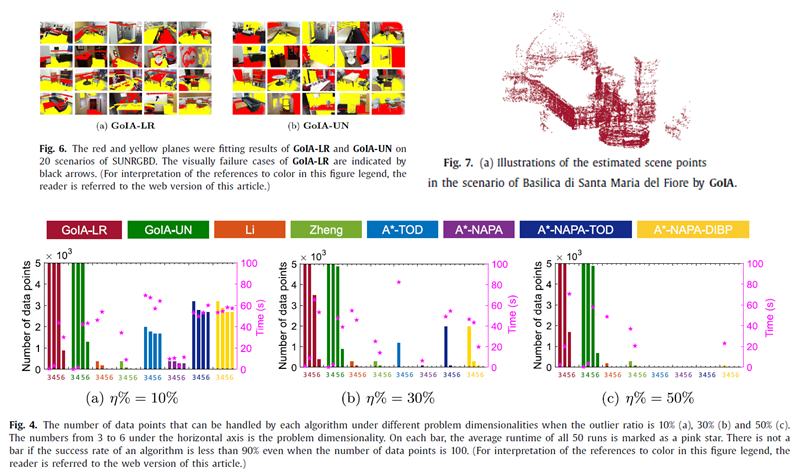Practical globally optimal consensus maximization by Branch-and-bound based on interval arithmetic

Practical globally optimal consensus maximization by Branch-and-bound based on interval arithmetic

Yiru Wang, Yinlong Liu, Xuechen Li, Chen Wang,   Manning Wang*, Zhijian Song*

Pattern Recognition（2021）

Abstract

Consensus maximization is widely used in robust modelfitting, and it is usually solved by RANSAC -type   methods   in   practice.   However, these   methods   cannot guarantee global optimality and sometimes return the wrongsolutions. A series of Branch-and-bound (BnB)   based globally   optimal methodshave   been proposed, most of which involve deriving a complex bound. Intervalarithmetic was utilized to derive simple bounds for BnB in solving geometricmatching problems in 2003. However, this idea was somewhat forgotten in thecommunity because it seems natural that the simple interval arithmetic basedbounds might be worse than those elaborate bounds. Recently, some new globallyoptimal algorithms without using BnB were developed for consensus maximization,but they can only work with a small number of data points and low outlierratios. In this work, we draw the idea of simple bounds by interval arithmeticback on the map and demonstrate its practicability by making substantialextensions. Concretely, we give detailed derivation of solving robust modelfitting problems with both linear and quasi-convex residuals and proposepractical methods to use them under Unit-Norm constraint and in ahigh-dimensional problem. Extensive experiments show that the proposed methodcan handle practical problems with large number of data points and high outlierratios. It outperforms state-of-the-art global, RANSAC-type, and deterministicmethods in terms of both accuracy and efficiency in low-dimensional problems.